Connect with us

# Capital Budgeting Model: Risk-Return Relationship, Cost Of Capital Calculation, Capital Budgeting WorksheetPublished

on

High-risk ventures should have expectations of high returns; low risk ventures will be expected to have a lower rate of return. Both must be made attractive to investors. Below graph demonstrates the relationship between the risk and return expected for a new line, extending a current line, and the modification or change of a product line or cost reduction programs.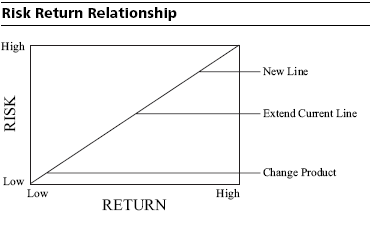From this relationship, a function can be derived to estimate the discount rate. The discount rate, or the necessary return for a project, is equal to the cost of capital plus the risk premium:

Required return = Cost of capital + Risk

The most important criterion in the calculation of a capital budgeting model is the required return. This number can be obtained by subjective estimation or by analytical methods that offer a means of estimating risk. But risk is mostly a perception in the mind of investors.

When considering the cost of capital to be used for the generation of net present value numbers, you should be concerned more with the incremental cost of capital for the project than with the overall cost of obtaining funds. It is the incremental cost of capital—the cost of financing this deal (internally or externally)—with which you are concerned when determining whether a project is cost justified. Nonetheless, it is also useful to know the company’s overall cost of capital, since each incremental funding decision will impact it. The next section describes how to calculate the cost of capital.

Components of the Cost of Capital

The components of the cost of capital are debt, preferred stock, and common stock. The least expensive of the three forms of funding is debt, followed by preferred stock and then common stock. Here we show how to calculate the cost of each of these three components of capital and then how to combine them into the weighted cost of capital.

When calculating the cost of debt, the key issue is that the interest expense is tax deductible“. This means that the tax paid by the company is reduced by the tax rate multiplied by the interest expense. This concept is shown in the next example, where we assume that \$1,000,000 of debt has a basic interest rate of 9.5 percent and the corporate tax rate is 35 percent.

Calculating the Interest Cost of Debt, Net of Taxes

Example: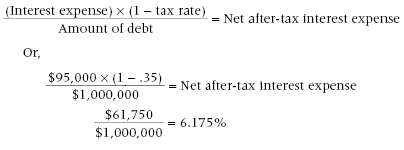The example clearly shows that the impact of taxes on the cost of debt significantly reduces the overall debt cost, thereby making this a most desirable form of funding. Preferred stock stands at a midway point between debt and common stock. The main feature shared by all kinds of preferred stock is that, under the tax laws, interest payments are treated as dividends instead of interest expense, which means that these payments are not tax deductible. This is a key issue, for it greatly increases the cost of funds for any company using this funding source. By way of comparison, if a company has a choice between issuing debt or preferred stock at the same rate, the difference in cost will be the tax savings on the debt. In the next example, a company issues \$1,000,000 of debt and \$1,000,000 of preferred stock, both at 9 percent interest rates, with an assumed 35 percent tax rate.

Debt cost = Principal × (interest rate × (1 – tax rate))
Debt cost = \$1,000,000 × (9% × (1 – .35))
\$58,500 = \$1,000,000 × (9% × .65)

If the same information is used to calculate the cost of payments using preferred stock, we have this result:

Preferred stock interest cost = Principal × interest rate
Preferred stock interest cost = \$1,000,000 × 9%
\$90,000 = \$1,000,000 × 9%

This example shows that the differential caused by the applicability of taxes to debt payments makes preferred stock a much more expensive alternative. The most difficult cost of funding to calculate by far is common stock, because there is no preset payment from which to derive a cost. One way to determine its cost is the capital asset pricing model (CAPM). This model derives the cost of common stock by determining the relative risk of holding the stock of a specific company as compared to a mix of all stocks in the market.

This risk is composed of three elements: The first is the return that any investor can expect from a risk-free investment, which usually is defined as the return on a government security. The second element is the return from a set of securities considered to have an average level of risk. This can be the average return on a large “market basket” of stocks, such as the Standard & Poor’s 500, the Dow Jones Industrials, or some other large cluster of stocks. The final element is a company’s beta, which defines the amount by which a specific stock’s returns vary from the returns of stocks with an average risk level. This information is provided by several of the major investment services, such as Value Line. A beta of 1.0 means that a specific stock is exactly as risky as the average stock, while a beta of 0.8 would represent a lower level of risk and a beta of 1.4 would be higher. When combined, this information yields the baseline return to be expected on any investment (the risk-free return), plus an added return that is based on the level of risk that an investor is assuming by purchasing a specific stock.

The calculation of the equity cost of capital using the CAPM methodology is relatively simple, once all components of the equation are available. For example: If the risk-free cost of capital is 5 percent, the return on the Dow Jones Industrials is 12 percent, and Royal Bali Cemerlang’s beta is 1.5, the cost of equity for Royal Bali Cemerlang would be:

Cost of equity capital = Risk-free return + Beta (Average stock return – risk free return)
Cost of equity capital = 5% + 1.5 (12% – 5%)
Cost of equity capital = 5% + 1.5 × 7%
Cost of equity capital = 5% + 10.5%
Cost of equity capital = 15.5%

Although the example uses a rather high beta that increases the cost of the stock, it is evident that, far from being an inexpensive form of funding, common stock is actually the most expensive, given the size of returns that investors demand in exchange for putting their money at risk with a company.

Now that we have derived the costs of debt, preferred stock, and common stock, it is time to assemble all three costs into a “Weighted Cost of Capital“. This section is structured in an example format, showing the method by which the weighted cost of capital of the Royal Bali Cemerlang Corporation is calculated.

The chief financial officer of the Royal Bali Cemerlang Corporation, Mr. Lie, is interested in determining the company’s weighted cost of capital, to be used to ensure that projects have a sufficient return on investment, which will keep the company from going to seed.

There are two debt offerings on the books:

The first is \$1,000,000 that was sold below par value, which garnered \$980,000 in cash proceeds. The company must pay interest of 8.5 percent on this debt. The second is for \$3,000,000 and was sold at par, but included legal fees of \$25,000. The interest rate on this debt is 10 percent. There is also \$2,500,000 of preferred stock on the books, which requires annual interest (or dividend) payments amounting to 9 percent of the amount contributed to the company by investors.

Finally, there is \$4,000,000 of common stock on the books. The risk-free rate of interest, as defined by the return on current government securities, is 6 percent, while the return expected from a typical market basket of related stocks is 12 percent. The company’s beta is 1.2, and it currently pays income taxes at a marginal rate of 35 percent. What is the Royal Bali Company’s weighted cost of capital?

The method we will use is to separate the percentage cost of each form of funding and then calculate the weighted cost of capital, based on the amount of funding and percentage cost of each of the above forms of funding. We begin with the first debt item, which was \$1,000,000 of debt that was sold for \$20,000 less than par value, at 8.5 percent debt. The marginal income tax rate is 35 percent.

The calculation is: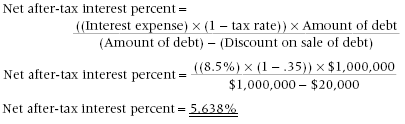We employ the same method for the second debt instrument, for which there is \$3,000,000 of debt that was sold at par. Legal fees of \$25,000 are incurred to place the debt, which pays 10 percent interest. The marginal income tax rate remains at 35 percent. The calculation is: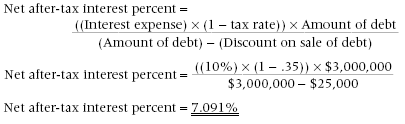Having completed the interest expense for the two debt offerings, we move on to the cost of the preferred stock. As noted, there is \$2,500,000 of preferred stock on the books, with an interest rate of 9 percent. The marginal corporate income tax does not apply, since the interest payments are treated like dividends and are not deductible. The calculation is the simplest of all, for the answer is 9 percent, since there is no income tax to confuse the issue.

To arrive at the cost of equity capital, we take from the example a return on risk-free securities of 6 percent, a return of 12 percent that is expected from a typical market basket of related stocks, and a beta of 1.2. We then plug this information into the formula to arrive at the cost of equity capital:

Cost of equity capital = Risk-free return + Beta (Average stock return – risk free return)
Cost of equity capital = 6% + 1.2 (12% – 6%)
Cost of equity capital = 13.2%

Now that we know the cost of each type of funding, it is a simple matter to construct a table listing the amount of each type of funding and its related cost, which we can quickly sum to arrive at a weighted cost of capital. The weighted cost of capital is 9.75 percent.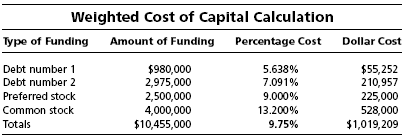Capital Budgeting Worksheet

Below is a common “Capital Budgeting Worksheet”, have a look the worksheet: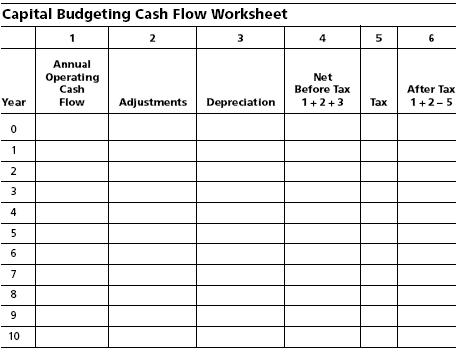How To Fill Out The Capital Budgeting Worksheet?

. Column 1 is used to list the estimated net annual cash inflows and outflows. Misestimating or underestimating in early years is more damaging than incorrect estimates of amounts in the distant future as a result of the discount factor. Thus, greater significance is placed on cash flows in the beginning periods. The initial outflow usually will be in year zero, which means as of Day 1 of the project period. Therefore, the discount factor is 1.0000 because that is current dollars. (Remember that for most discounting tables, all cash flows are assumed to occur at the end of each year. Although this may be an unrealistic assumption in that carrying costs may be incurred throughout the year, these carrying costs can be calculated and added to the net cash outflows to predict more accurately the total first year cost).

The initial investment includes not only the usual items, such as plant and equipment, but also investments in inventory, accounts receivable, training, product introduction, and the expenses for administrative changes and accounting. The next section provides a more detailed list of cash flow items to check in the capital budgeting proposals.

For new products, annual inflows that stay level without fluctuations should usually be suspect because the actual patterns seldom occur this way.

. In column 2, cash adjustments should include such items as buildups of accounts receivable and inventories. This allows recognition of the actual cash flows in appropriate years. For example, a new product line may build up \$500,000 in inventories in the first year, which may not be recovered in cash inflow until the end of the product’s life cycle. Taxes and the treatment of expenses and income for tax purposes must be considered and adjusted for in the model. For example, increases in receivables and inventories are examples of adjustments that affect current tax liabilities and must be considered in the calculation of estimated taxes. Inventories require cash in the year purchased but have tax effects when used or sold. Receivables may be taxable in the year sales are made, even though not collected until later periods.

Also, the effect of investment tax credits and other project related tax deductions should be included for the period in which the cash impact occurs.

. Depreciation is included solely for the purpose of considering its effect on taxes. The model uses only cash flows and the items affecting cash flows. Depreciation expense is a non-cash item in the current period. If depreciation has already been “expensed” in the operating cash flows of column 1, then an adjustment is necessary to ensure that it is not double counted. In the model, the full cost of the investment is made in period 0. Showing the allocation of that cost again through depreciation will count it twice.

. Column 4 calculates the taxable portion of the inflows. Care must be taken to determine the appropriate tax consequences, as the goal of the model is to determine after-tax cash flows.

. Column 5 is used for the calculation of tax, which must be subtracted from cash flows.

. Column 6 is the after-tax cash flow to be used in the various capital budgeting models for the evaluation of the proposals.

Capital Budgeting

Capital Budget Evaluation – Cash Flow and Capital Budget Proposal

Capital Budgeting Evaluation Worksheet – Improving Estimates

Comparison of the Internal Rate of Return (IRR) and Net Present Value (NPV) Method

Are you looking for easy accounting tutorial? Established since 2007, Accounting-Financial-Tax.com hosts more than 1300 articles (still growing), and has helped millions accounting student, teacher, junior accountants and small business owners, worldwide.